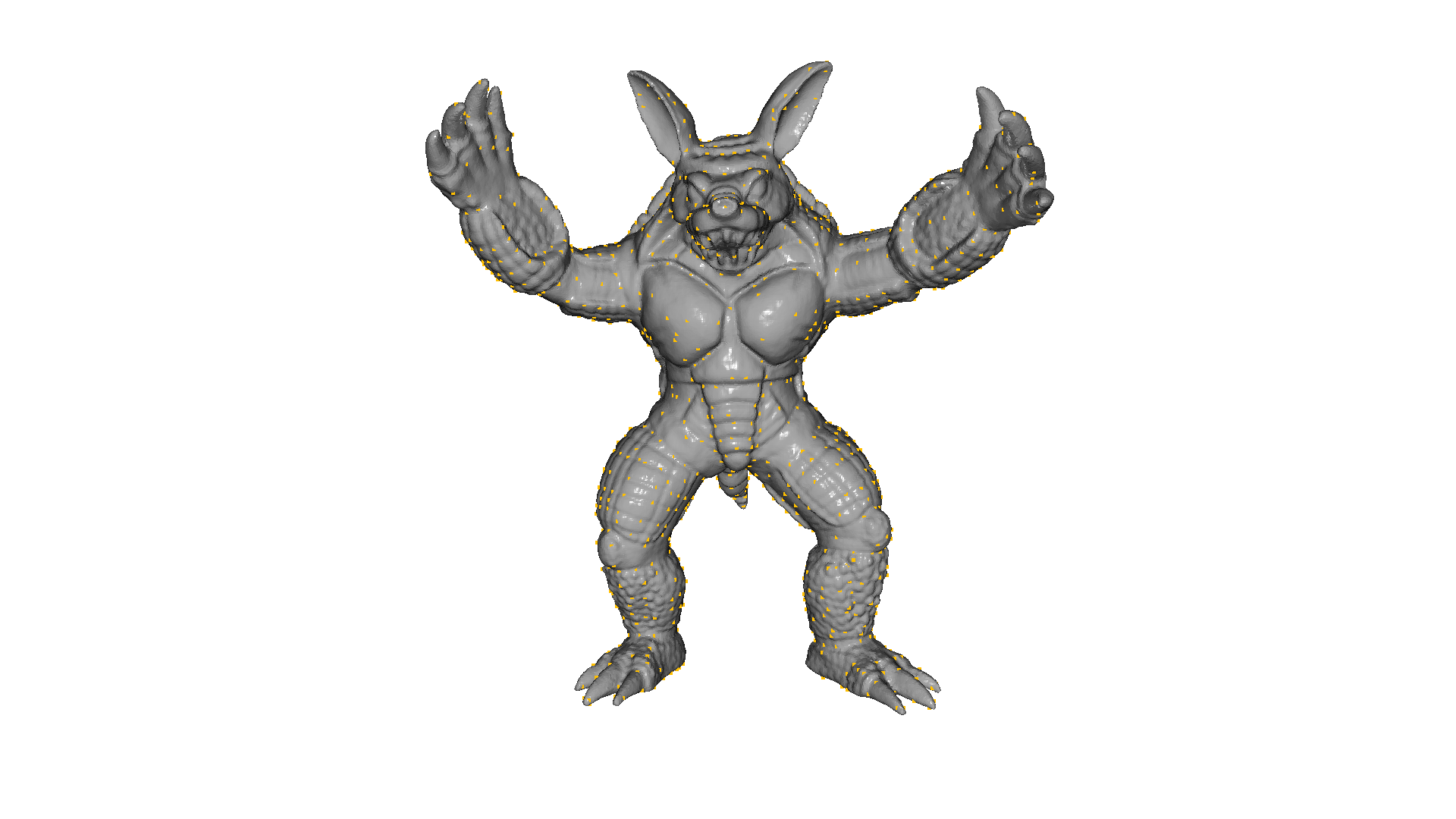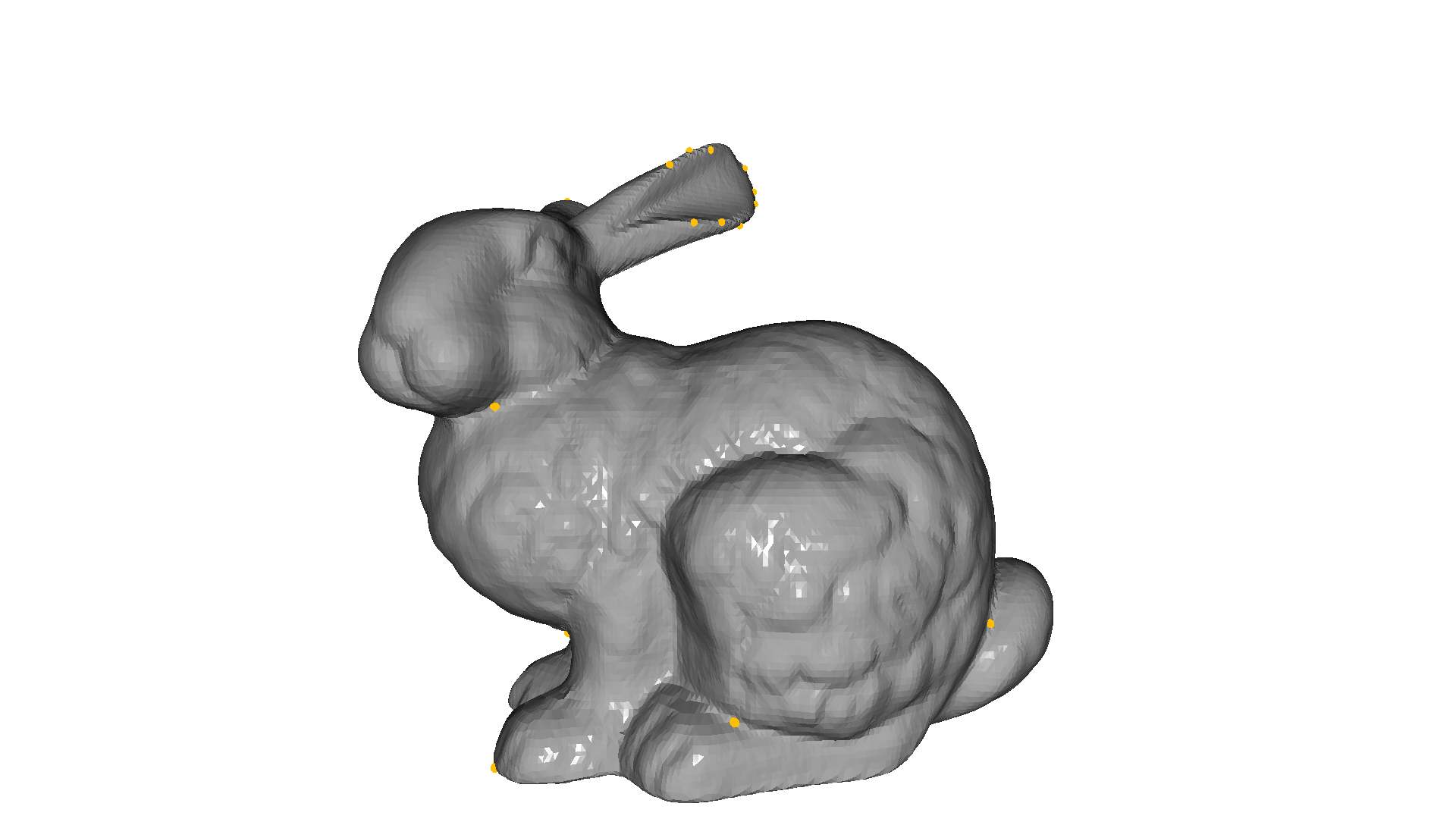# Intrinsic shape signatures (ISS)#

In this tutorial we will show how to detect the ISS Keypoints of a 3D shape. The implementation is based on the keypoint detection modules proposed in Yu Zhong , “Intrinsic Shape Signatures: A Shape Descriptor for 3D Object Recognition”, 2009.

## ISS Keypoints#

ISS saliency measure is based on the Eigenvalue Decomposition (EVD) of the scatter matrix $$\boldsymbol{\Sigma}(\mathbf{p})$$ of the points belonging to the support of $$p$$, i.e.

$\begin{split}\begin{array}{l} \boldsymbol{\Sigma}(\mathbf{p})=\frac{1}{N} \sum_{\mathbf{q} \in \mathcal{N}(\mathbf{p})}\left(\mathbf{q}-\mu_{\mathbf{p}}\right)\left(\mathbf{q}-\mu_{\mathbf{p}}\right)^{T} \quad \text { with } \\ \mu_{\mathbf{p}}=\frac{1}{N} \sum_{\mathbf{q} \in \mathcal{N}(\mathbf{p})} \mathbf{q} \end{array}\end{split}$

Given $$\boldsymbol{\Sigma}(\mathbf{p})$$, its eigenvalues in decreasing magnitude order are denoted here as $$\lambda_1$$, $$\lambda_2$$, $$\lambda_3$$. During the pruning stage, points whose ratio between two successive eigenvalues is below a threshold are retained:

$\frac{\lambda_{2}(\mathbf{p})}{\lambda_{1}(\mathbf{p})}<\gamma_{12} \wedge \frac{\lambda_{3}(\mathbf{p})}{\lambda_{2}(\mathbf{p})}<\gamma_{23}$

The rationale is to avoid detecting keypoints at points exhibiting a similar spread along the principal directions, where a repeatable canonical reference frame cannot be established and, therefore, the subsequent description stage can hardly turn out effective. Among remaining points, the saliency is determined by the magnitude of the smallest eigenvalue

$\rho(\mathbf{p}) \doteq \lambda_{3}(\mathbf{p})$

So as to include only points with large variations along each principal direction.

After the detection step, a point will be considered a keypoint if it has the maximum saliency value on a given neighborhood.

NOTE: For more details please refer to the original publication or to “Performance Evaluation of 3D Keypoint Detectors”, by Tombari et.al.

## ISS keypoint detection example#

:

# Compute ISS Keypoints on ArmadilloMesh
mesh.compute_vertex_normals()

pcd = o3d.geometry.PointCloud()
pcd.points = mesh.vertices

tic = time.time()
keypoints = o3d.geometry.keypoint.compute_iss_keypoints(pcd)
toc = 1000 * (time.time() - tic)
print("ISS Computation took {:.0f} [ms]".format(toc))

mesh.compute_vertex_normals()
mesh.paint_uniform_color([0.5, 0.5, 0.5])
keypoints.paint_uniform_color([1.0, 0.75, 0.0])
o3d.visualization.draw_geometries([keypoints, mesh], front=[0, 0, -1.0])

ISS Computation took 652 [ms]:

# This function is only used to make the keypoints look better on the rendering
def keypoints_to_spheres(keypoints):
spheres = o3d.geometry.TriangleMesh()
for keypoint in keypoints.points:
sphere.translate(keypoint)
spheres += sphere
spheres.paint_uniform_color([1.0, 0.75, 0.0])
return spheres

:

# Compute ISS Keypoints on Standford BunnyMesh, changing the default parameters
bunny = o3d.data.BunnyMesh()
mesh.compute_vertex_normals()

pcd = o3d.geometry.PointCloud()
pcd.points = mesh.vertices

tic = time.time()
keypoints = o3d.geometry.keypoint.compute_iss_keypoints(pcd,

ISS Computation took 56 [ms]